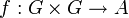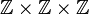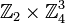# Group of IIP 2-cocycles for trivial group action is naturally identified with group of homomorphisms from a particular abelian group

## Statement

Suppose$G$ is a group. Then, there exists an abelian group$K$ such that, for any abelian group$A$, the group$Z^2_{IIP}(G,A)$ of IIP 2-cocycles$\! f:G \times G \to A$ for the trivial group actioncan be identified with the group of homomorphisms$\operatorname{Hom}(K,A)$ under pointwise addition.

## Examples

Group$G$ Group$K$ that acts as source of homomorphisms
cyclic group:Z4 group of integers
Klein four-group cyclic group:Z4
cyclic group:Z8$\mathbb{Z} \times \mathbb{Z} \times \mathbb{Z}$
direct product of Z4 and Z2$\mathbb{Z}_2 \times \mathbb{Z}_2 \times \mathbb{Z} \times \mathbb{Z}$
elementary abelian group:E8 direct product of Z4 and Z4 and Z4 and Z2 ($\! \mathbb{Z}_2 \times \mathbb{Z}_4^3$)
cyclic group:Z16$\! \mathbb{Z}^7$
direct product of Z4 and Z4$\! \mathbb{Z}^6 \times \mathbb{Z}_2 \times \mathbb{Z}_4$
direct product of Z8 and Z2$\! \mathbb{Z}_2^2 \times \mathbb{Z}^6$
direct product of Z4 and V4$\! \mathbb{Z}_2^5 \times \mathbb{Z}_4 \times \mathbb{Z}^4$
elementary abelian group:E16$\! \mathbb{Z}_2^5 \times \mathbb{Z}_4^6$
elementary abelian group:E32$\! \mathbb{Z}_2^{16} \times \mathbb{Z}_4^{10}$